BASH Programming

# Bash Scripting Tutorial for Beginners

The default command language of Linux is Bash script. We need to run many commands in Linux on a daily basis for many purposes. These daily tasks can be automated by using a bash script. Any user can learn this scripting language very easily and quickly. If you are new in bash programming then this tutorial is for you.

## Contents:

Adding comments with the code is an essential part of any programming language. The comments are not parsed with the script at the time of execution. The reader can understand any code properly if the code is well commented. You can add a comment in multiple ways in bash script. How you can add single-line and multiple-lines comments in a bash script is shown in this part. Create a bash file with the following script to know the use of bash comment. Here, ‘#’ symbol is used for adding a single-line comment and single quote (‘) with ‘:’ is used for adding multiple-lines comments.

bash_comment.sh

#!/bin/bash
#Take a number as input
echo "Enter a number"
: '
Check the input number is
less than 10 or greater than 10 or equal to 10
'

if [[ \$a -lt 10 ]]
then
echo "The number is less than 10"
elif [[ \$a -gt 10 ]]
then
echo "The number is greater than 10"
else
echo "The number is equal to 10"
fi

Output:

Run the script.

\$ bash bash_comment.sh

Here, the script is executed for three times with the input value 3, 10 and 90. The following output will appear.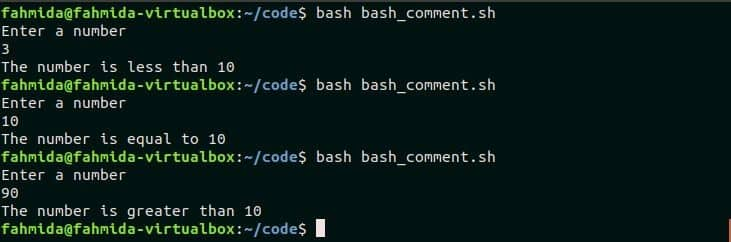If you want to know more about bash commenting then you can check this tutorial.

Go to Top

## echo command

`echo` command is used in bash to print output in the terminal. Various options can be used with echo command in bash to print the output in different ways. Create a bash file with the following script to know the two simple uses of `echo` command. Here, the first `echo` command will print a simple text data with a new line and second echo command will print a simple text without a newline.

echo_test.sh

#!/bin/bash
#Print the first text
echo "Print text with a new line"
#Print the second text
echo -n "Print text without a new line"

Output:

Run the script.

\$ bash echo_test.sh

The following output will appear after executing the above command.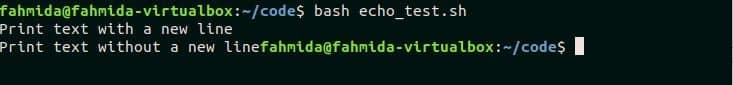There are many other uses of `echo` command in bash. You can check this tutorial to know more about `echo` command.

Go to Top

### Variables

Variable declaration is a necessary part of any programming language. Bash variables can be declared in different ways. When a value is assigned in a variable then no symbol is used at the beginning of the variable. ‘\$’ symbol is used with the variable name at the time of reading the value of the variable. Variable can be used from the terminal or can be used in any bash script.

The following commands will declare a string variable named mystr with a particular value and next print the value of the variable in the terminal.

\$ mystr="I like bash programming"
\$ echo \$mystr

Output: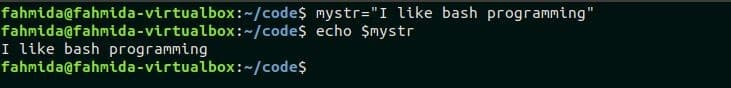Create a bash file with the following script. Two variables are declared here. These are \$a and \$b. If the input value which is stored in \$a is equal to \$b then the message,  “Numbers are equal” will be printed otherwise “Numbers are not equal” will be printed.

var.sh

#!/bin/bash
echo "Enter a number"
b=100
if [[ \$a -eq \$b ]]
then
echo "Numbers are equal"
else
echo "Numbers are not equal"
fi

Output:

Run the script.

\$ bash var.sh

The above command is executed for two times with the value 56 and 100. The following output will appear.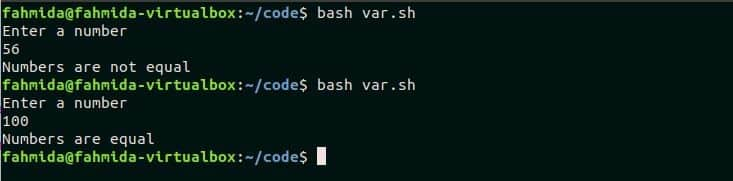If you want to know more about bash variables then you can check this tutorial.

Go to Top

### Conditional statement

Like another programming language, you can use conditional statement in bash. ‘If-then-else’ and ‘case’ statements are mainly used for implementing condition statements in any programming language. The use of conditional statement by using ‘if’ statement is shown in this section of this tutorial. Create a bash file with the following script where conditional statement is used. Here, two values will be taken from the user as input and stored in the variables, \$code, and \$age. ‘if’ statement is used to check the value of \$age is greater than or equal to 18 and the value of the \$code is 1100. If both conditions are true then the message, “You are eligible to see the movie” will be printed otherwise “You are not eligible to see the movie” will be printed.

cond.sh

#!/bin/bash

if [[ \$age -ge 18 && \$code -eq '1100' ]]
then
echo "You are eligible to see the movie"
else
echo "You are not eligible to see the movie"
fi

Output:

Run the script.

\$ bash cond.sh

The following output will appear after taking different input values. 1100 is given as a code and 5 is given as age for the first execution and if condition returns false for these values. 1100 is given as a code and 45 is given as age for the second execution that returns true for if condition.If you want to know more about bash conditional statement then you can check this tutorial.

Go to Top

### Loops

When some parts of the script need to execute multiple times then the loop is used to do the task. Bash supports three types of loops declaration like other languages. These are for, while and until loops. Based on the programming requirements, a particular loop is used in the script. The uses of these three types of loops are shown in this section by using simple examples.

#### Using for loop

Create a bash file with the following script where `for` loop is used for iteration. `for` loop is mainly used for iterating a list of data or an array. Here, a list of weekday name is used and each weekday name is iterated by using for loop. `if` statement is used to print a particular message based on weekday name.

for.sh

#Read a weekday name in each iteration of the loop
for day in Monday Tuesday Wednesday Thursday Friday Saturday Sunday
do

#Check the weekday name is Monday or Thursday
if [[ \$day == 'Monday'  || \$day == 'Thursday' ]]
then
echo "Meeting on \$day at 9:30 am"

#Check the weekday name is Tuesday or Wednesday or Friday
elif [[ \$day == 'Tuesday'  || \$day == 'Wednesday' || \$day == 'Friday' ]]
then
echo "Training on \$day at 11:00 am"
else

#Print ‘Holiday’ for other days
echo "\$day is Holiday"
fi
done

Output:

Run the script.

\$ bash for.sh

The following output will appear after running the script.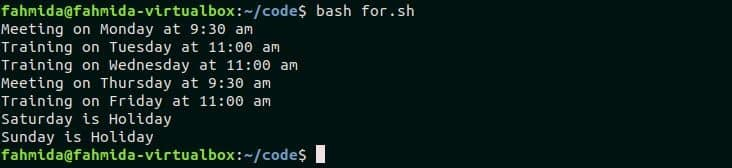If you want to know more used of bash for loop then you can check this tutorial.

#### Using while loop

Create a bash file with the following script where `while ` loop is used for iteration. This script will print those numbers from 1 to 20 which are even and divisible by 5. Here, \$counter variable is used to control the iteration of the loop and the value of this variable is incremented by 1 in each iteration. When `if` condition will return true then it will print the value of \$counter.

#!/bin/bash

#Print the message
echo "Print the numbers which are even and divisible by 5"

#Initialize the counter
counter=1

#Iterate the loop until the \$counter value is less than or equal to 20
while [ \$counter -le 20 ]
do

#Check the \$counter is divisible by 2 and 5
if [[ \$counter%2 -eq 0 && \$counter%5 -eq 0 ]]
then
#Print \$counter without newline
echo  "\$counter"
fi

#Increment \$counter by 1
((counter++))
done
echo "Done"

Output:

\$ bash while.sh

There are only numbers within 1-20 those are even and divisible by 5. The following output will appear after running the script.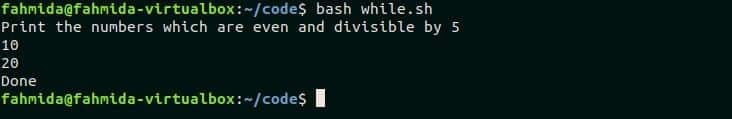If you want to know more used of bash `while` loop then you can check this tutorial.

#### Using until loop

Create a bash file with the following script where `until` loop is used for iteration. This script will print all odd numbers from 0 to 20. \$n variable is used in this script to iterate the loop.

until.sh

#!/bin/bash

#Initialize the variable, n
n=20

#Repeat the loop until the value of \$n is greater than 0
until [ \$n -lt 0 ]
do

#Check the value of n is odd
if [[ \$n%2 -gt 0 ]]
then
echo \$n
fi

#Increment the value of n by 1
((n=\$n-1))
done

Output:

Run the script.

\$ bash until.sh

The script will print all even numbers from 20 to 1. The following output will appear after running the script.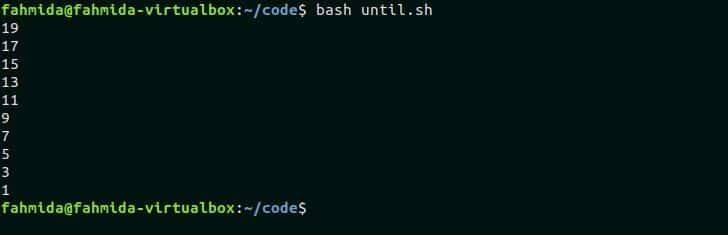Go to Top

### Functions

When a block of code needs to execute multiple times in a script then the function is used to do the task. You will need to call the function by name only when the script defined in the function need to execute many times rather than adding the same script multiple times. The starting and ending first bracket is used with function name to declare the function in bash script. The function can be called by just the function name in bash. Bash does not support function argument like another standard programming language. But the value can be passed to bash function in a different way that is shown in this section. The value can be returned from the function with the return statement or without using the return statement.

Create a bash file with the following script to know how the functions can be declared and called in bash script. Three functions are declared in the script. The first function is declared to print a simple message, “Bash Programming for beginner”. The second function is declared to assign a string value in a variable, \$return_str that will print,  “Learn bash programming with LinuxHint” after calling the function. The third function is declared to read an argument value as circle radius that will be provided at the time of calling the function. Here, local is used to read the argument value. This function will calculate the area of the circle based on radius value by using the formula, πr2 and print the calculated area value.

func.sh

#!/bin/bash

#Declare a simple function
function print_message()
{
echo "Bash programming for beginner"
}

#Declare a function to return a string value
function ret_strdata()
{
#Initialize the variable with string value
return_str="Learn bash programming with LinuxHint"
}

#Declare a function to read argument value
function calculate_area()
{
#Print the area value
echo "Area of the circle is \$area"
}

#Call the function to print a simple message
print_message

#Call the function that will assign a string value in a variable
ret_strdata

#Print the value of the variable
echo \$return_str

#Call the function with radius value

Output:

Run the script.

\$ bash func.sh

The following output will appear after running the script. The first two lines will print by calling the functions, print_message() and ret_strdata(). The last line will print by calling the function, calculate_area() with the taken input radius value.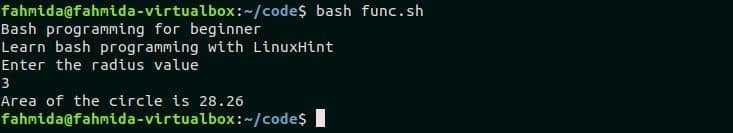If you want to know about returning a string from bash function then you can this tutorial.

Go to Top

### Calling programs in a script

You can use many types of commands to call other programs in any bash script, such as source, bash, eval, exec, etc. Suppose three bash files, add.sh, subtract.sh,  multiply.sh and division.sh created to perform addition, subtraction, multiplication, and division. Here, subtract.sh and division.sh read command line arguments. The script of all these four files is given below.

#!/bin/bash
a=60
b=40
((result=\$a+\$b))

subract.sh

#!/bin/bash
a=\$1
b=\$2
((result=\$a-\$b))
echo "The subtraction of \$a-\$b=\$result"

multiply.sh

#!/bin/bash
((result=\$1*\$2))
echo "The multiplication of \$1 and \$2 is \$result"

divide.sh

#!/bin/bash
a=\$1
b=2
((result=\$a/\$b))
echo "The division of \$a by \$b is \$result"

Create a bash file named, callpro.sh with the following script to call the mentioned bash files using source, bash, eval and exec commands. You must set execution permission for the above four files before running the following script. `source` command is used to call add.sh file. `bash` command is used to execute subtract.sh file. `eval` command is used to execute multiply.sh file. Two input values are sent as command line arguments for `eval` command. The last command is exec command that works with absolute path only. For this, the full path name of divide.sh file is given in the script.

callpro.sh

#!/bin/bash
script2="subtract.sh"
script3="multiply.sh"
script4="/home/fahmida/code/divide.sh"

source "\$script1"

bash \$script2 50 20

echo "Enter the value of a"
echo "Enter the value of b"
eval bash \$script3 \$a \$b
exec \$script4 30

Output:

Run the script.

\$ bash callpro.sh

The following output will appear after running the script.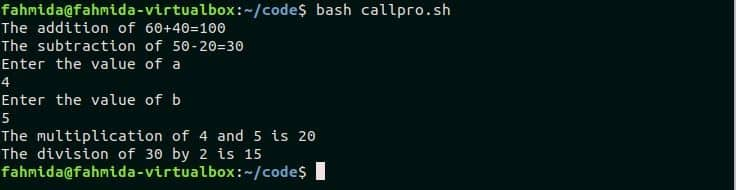Go to Top

There is a useful command in bash to create a simple menu which is called `select` command. Different types of menus can be created by using this command. A particular data list is used to create a menu by this command. Create a bash file with the following code to see the use of `select` command for creating a menu. In this example, the list of five items will be printed as a menu and prompt the user to choose any language from the list. The selected value will be stored in the variable, \$language that is printed later by concatenating with other string. The script will continuously ask for selecting the language until the user presses 6 to terminate from the script.

#!/bin/bash

#Print message for the user

# Define the list of a menu item
select language in C# Java PHP Python Bash Exit
do
#Print the selected value
if [[ \$language == "Exit" ]]
then
exit 0
else
echo "Selected language is \$language"
fi
done

Output:

Run the script.

According to the following output, the user pressed 3 for the first time that printed PHP and pressed 6 for the second time that terminated from the script.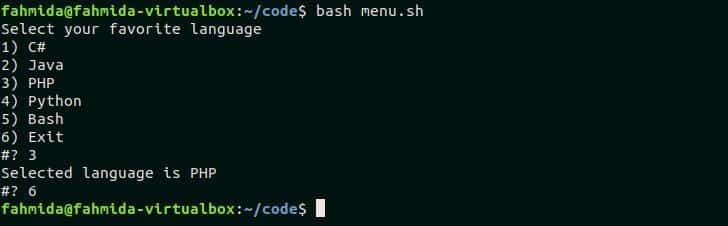If you want to know more about bash menu creation with `select` then you can visit this tutorial.

Go to Top

### Command Line Processing

Sometimes we need to provide input values when executing the script from the command line. This task can be done in two ways in bash. One way is by using argument variables and another way is by using getopts function. Reading command line input data from the terminal by using the argument variable is shown in this section.

Create a bash file with the following script to see the use of reading command line argument value. This script will read three command line arguments which will be stored in the variables, \$operand1, \$operand2 and \$operator. To execute the script properly, the first and third argument values must be the number and second argument value must be any of the four arithmetic operators (‘+’, ‘-’, ‘/’, ‘x’). if statement will check the value of \$operator and do the operation based on the operator and print the value.

cl1.sh

#!/bin/bash

#Print the argument variables
echo "Argument values are: \$1 \$2 \$3"

# Store argument values
operand1=\$1
operand2=\$3
operator=\$2

#Check the 2nd command argument value to do the arithmetic operation
if [[ \$operator == '+' ]]
then
((result=\$operand1+\$operand2))
elif [[ \$operator == '-' ]]
then
((result=\$operand1-\$operand2))
elif [[ \$operator == 'x' ]]
then
((result=\$operand1*\$operand2))
elif [[ \$operator == '/' ]]
then

((result=\$operand1/\$operand2))
fi

# print the result
echo -e "Result is = \$result"

Output:

Run the script.

\$ bash cl1.sh

The script is executed for four times for four types of arithmetic operators. The following output will appear for the argument values, 6 + 3, 6 – 3, 6 x 3 and 6 / 3.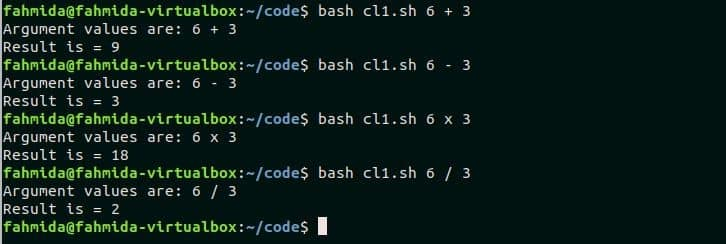Argument values can be passed with name-value pair in bash. Create a bash file with the following script to show how to read argument values with the name. The script will read two argument variables. The argument values with name are printed in the first statement of the script. Next, a for loop is used to iterate the array that contains command line argument values. Each element of the array is separated into a key-value pair by using `cut` command. Next, case statement is used to print a particular message based on the key value.

cl2.sh

.#!/bin/bash

#Print the argument variables
echo "Argument values are: \$1 \$2"

#Read each argument separately using for loop
for arg in "\$@"
do

#Separate argument name and value
key=\$(echo \$arg | cut -f1 -d=)
value=\$(echo \$arg | cut -f2 -d=)

#Print message based on argument’s name
case \$key in
name) echo "Student’s name = \$value";;
mark) echo "Obtained mark = \$value" ;;
*)
esac
done

Output:

Run the script with following command line arguments.

\$ bash cl2.sh name=”Abir Hossain” mark=90

Two command line arguments are provided in the above commands. These are name=”Abir Hossain” and mark=90. name and marks are separated by the script and two values are printed after formatting the output.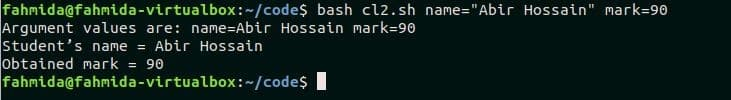Processing command line arguments by using getopts function is not discussed in this tutorial. If you want to know about command line processing by using getopts function then you can visit this tutorial.

Go to Top

### Arithmetic

Doing arithmetic operation is a common requirement of any programming language. Bash does the arithmetic operation in a different way than another standard programming language. There are multiple ways to do arithmetic operations in bash. One of the simple ways of doing the arithmetic operation is shown in this section. Create a bash file with the following script. Four types of arithmetic operations are shown in this script. A simple summation and division operations are shown by using double first brackets at the beginning of the script. Next, the pre-increment operation is shown. Finally, the way to use shorthand operator is shown in the last part of the script.

arith.sh

#!/bin/bash
# Calculate the sum
result=\$((50+25))
# Print summation value
echo "sum = \$result"

# Calculate the division
result=\$((50/25))
# Print division value
echo "division = \$result"

# Assign a value to N
N=10
# Doing pre-increment
((--N))
# Print the value of N
echo "Value after decrement = \$N"

# Using shorthand operator
(( N += 10 ))
# Print the value of N
echo "Value after adding 10 = \$N"

Output:

Run the script.

\$ bash arith.sh

The following output will appear after running the script.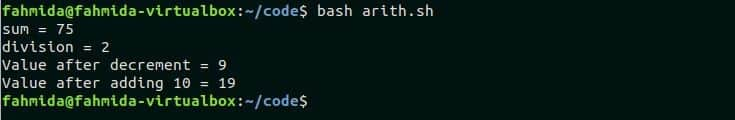All arithmetic operations are done by using double brackets in this above script. But you can use ‘let’, ‘expr’ and ‘bc‘ command to do the arithmetic operation in bash. If you want to know more about these commands for doing bash arithmetic operations then you can visit this tutorial.

Go to Top

### String Manipulation

Many types of tasks can be done with the string data in bash. Some are concatenating string, comparing string, splitting a string, Changing case of the string, etc. There are no built-in string functions like other standard languages in bash to do the string operations. Some common string manipulations are discussed in this section of this tutorial.

#### Concatenating String

Adding two or more strings together is called string concatenation. The string is joined together in bash by placing one after another. Create a bash file with the following script to show the use of string concatenation. Two string variables are initialized and print after combining the variables. Here, the content of \$string1 and \$string2 will be merged and printed.

concat.sh

#!/bin/bash

#Initialize first string variable
string1="I like "
#Initialize second string variable
string2="Bash Programming"
#Print after combining both strings
echo "\$string1\$string2"

Output:

Run the script.

\$ bash concat.sh

The following output will appear after running the script.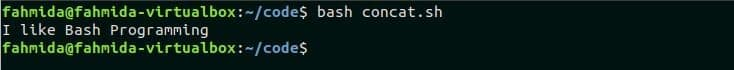You can know more about string concatenation from this tutorial.

Go to Top

### Comparing String

Bash uses different types of operators to compare string data. Create a bash file with the following script to show how to compare two string data. A string value is taken as input in the script that is compared with another string. If the value matches then a message, “You like Python” will be printed otherwise “You like PERL” will be printed.

compare.sh

#!/bin/bash
echo “Enter any string value”

#Check the input data is equivalent to “Python”
if [ \$text == "Python" ]; then
echo "You like Python."
else
echo "You like PERL"
fi

Output:

Run the script.

\$ bash compare.sh

The following output will appear after running the script where the input value is ‘PERL’.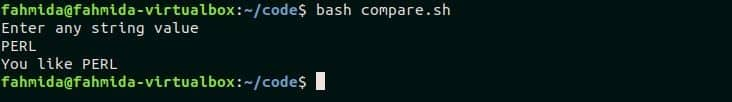You can know more about string comparison from this tutorial.

#### Splitting String

Bash does not have any built-in split function to divide string data. The string data can be divided by multiple ways in bash based on different types of delimiters. Create a bash file with the following script to show how string data can be divided into bash. A string value is taken as input. This script will divide the value of \$text based on space. Here, the IFS variable is used to set the delimiter. `read` command is used here to divide the text value and store the values into an array. for loop is used to iterate the array and print the value of each element.

split.sh

#!/bin/bash
#Input a string value
echo “Enter a string value”
# Set the delimiter
IFS=' '
#Split the value of \$text into an array based on space delimiter
# Print each value of the array
for value in "\${arr[@]}";
do
printf "\$value\n"
done

Output:

Run the script.

\$ bash split.sh

The following output will appear after taking the input, “Learn Bash programming”. This input value is a text of three words. So, the string is divided into three parts.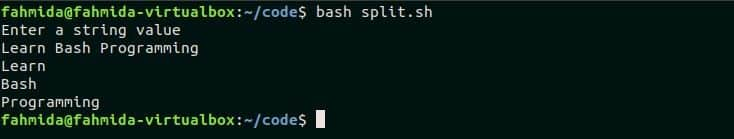You can know more about string comparison from this tutorial.

#### Changing Case of the string

Most of the scripting languages have built-in functions to change the case of the string data. But the case of the string data can be changed in bash by using `tr` command or by using ‘:upper’ and ‘:lower’ keywords. Create a bash file with the following script to know the ways of changing case in bash. Here, the first string data is converted to uppercase by using ‘^^’ symbol and the second string is converted to lowercase by using `tr` command. `tr` command will search all uppercase letter in the string and convert the characters into lowercase.

case.sh

#!/bin/bash

#Initialize the first string data
text1='test@example.com'

#Print the value of \$text1 by converting all characters to uppercase
echo\${email^^}

#Initialize the second string data
text2=’Bash Programming Basics’

#Print the value of \$text2 by converting all uppercase to lowercase
echo \$text2 | tr [:upper:] [:lower:]

Output:

Run the script.

\$ bash case.sh

The following output will appear after running the script.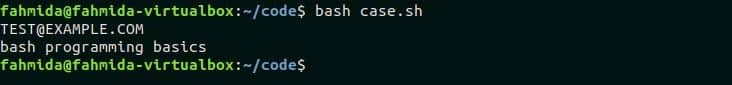You can know more about string comparison from this tutorial.

Go to Top

#### Reading string data through the loop

The string data works as a character array for any programming language. How ‘for’ loop can be used to read string data in bash is shown in this section. Create a base file with the following script to read each part of the string value by using for loop.

#!/bin/bas
# Read each word of a text by using for loop
for value in Bash Programming for the Beginners
do
echo \$value
done

Output:

Run the script.

The following output will appear after running the script.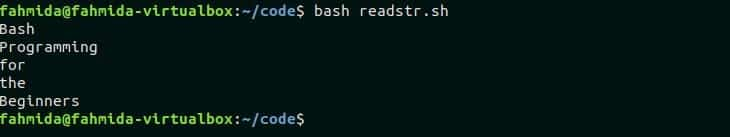You can know more about iterating string data by using the loop from this tutorial.

Go to Top

### Returning return codes from a script and catching in another script

One bash script can catch return codes from another script by calling the script and using ‘\$?’ to read the return value. Suppose, a bash file named first.sh returns a code after execution. Create another bash file named second.sh and add the following script to catch the return value and do some other tasks. The code of both files is given below. first.sh file will be called from second.sh file at the beginning of the script. first.sh will return an exit code based on the input value. second.sh will catch the code by ‘\$?’ and compare with 1. If both values are equal then it will print, “The input number is greater than 100”, otherwise it will print, “The input number is less than or equal to 100“.

first.sh

#!/bin/bash

echo "Enter a numeric value"

# Check the input value is less than or equal to 100 or not
if [[ \$n -le 100 ]]
then
exit 0
else
exit 1
fi

second.sh

#! /bin/bash

#Execute the file, first.sh
bash "first.sh"

#Check the return code is equal to 1 or not
if [ \$? -eq 1 ]
then
echo "The input number is greater than 100"
else
echo "The input number is less than or equal to 100"
fi

Output:

Run the script.

\$ bash second.sh

The following output will appear when the script is executed by 55 and 110 for two times.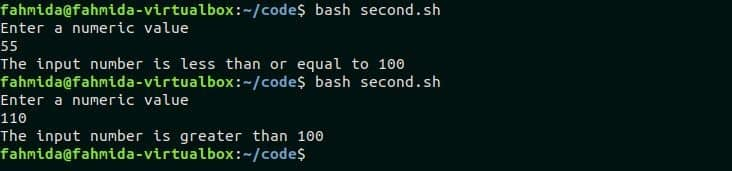Go to Top

### Reading and writing to files

Reading and writing files are common requirements of bash programming. Bash does not have any built-in function like another language to read or write the file. There are multiple ways to read the file in bash. The most common way to read or write a file in bash is using `cat` command. But this command is used to read the whole content of the file at a time. You can also read any file line by line by using any loop and `read` command. Using redirect operator, ‘>’, you can write data into any file in bash. If you want to append data into any file then you have to use ‘>>’ operator. Both reading and writing file operations are shown in the next part of this section.

Create a bash file with the following script to read an existing file named ‘hardware.txt’. The content of this file is given below. In the script, the whole content of the file is read by `cat` command first and next, while loop is used to read the file line by line.

hardware.txt

Monitor
Keyboard
Mouse
Scanner
Printer

#!/bin/bash

echo "Reading file using cat command"

# Read the content of the file using `cat` command
content=`cat hardware.txt`
echo \$content

echo "Reading file line by line using loop"

# Assign the filename
filename='hardware.txt'

# Each line of the file will be read by each iteration of the loop
do
# print the line
echo \$line
done<\$filename

Output:

Run the following commands.

\$ cat  hardware.txt

Here, the first command will print the content of the file, hardware.txt without running any bash script and the second command will run the script of readfile.sh  and print the content of the file for two times by using `cat` command and `read` command with while loop. You can visit this tutorial to know more about reading file line by line.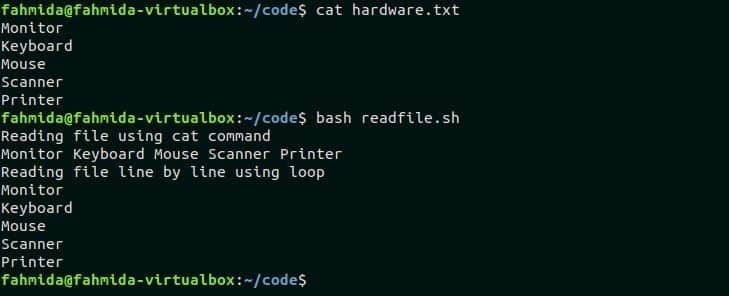#### Writing file in bash

Create a bash file with the following script to write new content in a new file and append data in that file.

writefile.sh

#!/bin/bash

echo "Enter some text"
#Add input data into the file for the first time
echo \$str1 > test.txt

echo "Enter some other text"
#Add input data at the end of the file
echo \$str2 >> test.txt

#Show the full content of the file
echo `cat test.txt`

Output:

Run the script.

\$ bash writefile.sh

The following output will appear after running the script.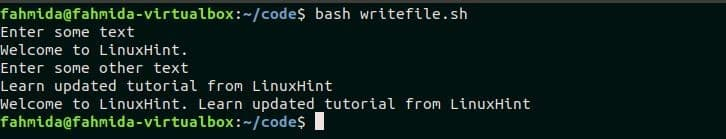Go to Top

### Pipes

Pipe is used to redirect any command output to other command input. It can be used among different types of bash commands to generate specific output. The standard input is connected with standard output in Linux by pipe. You need to set the command in sequence with pipe ( | ) symbol to get the desired output. Two or more commands can be executed together in a single command by using pipe. You have to execute multiple commands in multiple lines without pipe to do the same task. So, using pipe is very beneficial for doing many types of tasks in a short form.

syntax:

command1 | command2 |

Here, the output of the command1 will be passed as the input of command2.

The same type of task is done with pipe and without a pipe in the next part of this section.  Suppose a text file named marks.txt is given with the following data.

marks.txt

Asraf   CSE-409         79

Kabir   CSE-304         95

Keya    CSE-101         67

Asraf   CSE-304         88

Keya    CSE-409         90

Asraf   CSE-101         92

You have to sort the data of the file and find out and print all the entry of the student name ‘Keya’. You can do this task by running multiple commands without using a pipe that is shown in the next section. The following commands will need to run to get the desired output. The first command will sort the file. The second command will search the entry ‘Keya’ using `grep` command and store the output in a temp.txt file. The third command will count the total lines of a temp.txt file by using `wc` command.

\$ sort marks.txt
\$ grep 'Keya' marks.txt > temp.txt
\$ wc -l temp.txt

Output:

Two entries of the student, ‘Keya’ exist in the file. So after running the above commands, the following output will appear.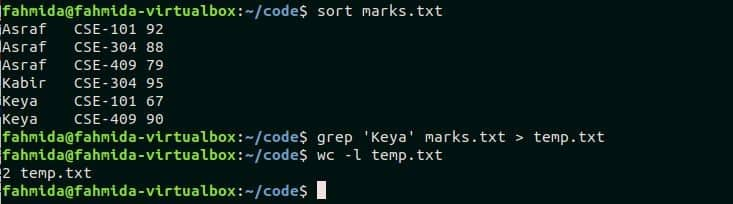You can easily merge the above three commands and get the same output by running a single command with a pipe that is shown in the following command. No temporary file is required here to get the output. Here, the output of the `sort` command will be passed as input of `grep` command and the output of the `grep` command will be passed as input for the `wc` command.

\$ sort marks.txt | grep 'Keya' | wc -l

Output:

Afttr running the above command you will get the following output like the previous command’s output. The output of the command will be 2.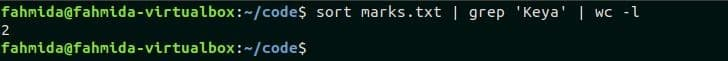Go to Top

#### Conclusion:

Most useful and necessary topics of bash scripting language are tried to cover in this tutorial. Hope, the reader will be benefited after reading this tutorial and able to write bash script more efficiently.